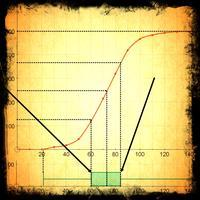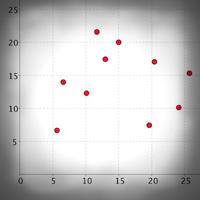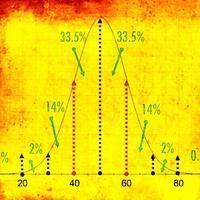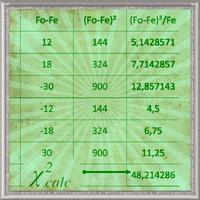# Statistics and Probability

##Data, data everywhere...

It seems like there has never been a time when more data was produced, collected, counted and processed. It follows perhaps that it has never been more important to understand and think carefully about the way it can be processed. It this section we explore everything from the subtle differences between a mean and a median, to bivariate data, outliers and on to the sophisticated concept of hypothesis testing and the significance of certain results. All this as well as the wonderful world of probability. Misunderstanding about lots of these ideas are often the root cause of misinformation. This is your chance to put that right!

## What is in this section?

#### 4.1 Statistical concepts

Key Concepts In this unit you should learn to… recognise the difference between discrete and continuous data be able to minimise the bias in your data take a sample of data in order to analyse it recognise difference types of sampling find outliers...

#### 4.2 & 4.3 Cumulative Frequency & Box Plots

Key Concepts In this unit you should learn to... text Essentials Look at the topic at a glance with these overview slides about cumulative frequency and box plots Mode: carousel Thumb width: 48px Layout: row This section offers a series of video...

#### 4.3 Central tendency and Dispersion

Mean, Mode, Median, Range, Variance, Standard Deviation Measures of central tendency, such as the mean, median and mode, are very useful ways of representing large amounts of data with just one value. They can be very useful in forming conclusions...

#### 4.4 Bivariate data & Linear Correlation

This topic is all about looking for relationships between variables. Does life expectancy depend on GDP? By collecting 2 variables about the things we survey we can use scatter graphs to represent the data and then look for correlation and...

#### 4.5, 4.6 Probability

Key Concepts In this unit you should learn to… recognise key terms calculate using sample space diagrams use tree diagrams find probabilities from tables and Venn diagrams use conditional probability Essentials Slides Gallery - Probability Part 1

#### 4.7 Discrete random variables

Random variables are a set of possible outcomes from a random experiment. They can either be discrete or continuous. In this chapter we will find out more about discrete random variables, how they can be represented, displayed and analysed.

#### 4.8 Binomial Distribution

Key Concepts In this unit you should learn to… understand what types of data are binomially distributed be familiar using binomial notation calculate the expected value, standard deviation and variance of a binomially distributed event calculate...

#### 4.9 Normal Distribution

The normal distribution is a fascinating, naturally occurring phenomenon that has very relevant applications to understanding the world around us. When a data set is normally distributed it has some key properties that allow us to make predictions...

#### 4.10 Spearman's Rank Correlation Coefficient

Analysing how closely two things are correlated is incredibly useful to ascertain exactly how strongly one variable affects another. Whilst helpful if the data is approximately linear, Pearson's Product Moment Correlation Coefficient can...

#### 4.11 Chi Squared Independence tests

The chi squared independence tests is a widely used technique for looking for a relationship between variables that are categorical. We can use a scatter graph to look for a relationship between GDP and life expectancy, but what about GDP and...

#### 4.11 Chi Squared Goodness of Fit and the T-Test

This page continues and extends our understanding of hypothesis testing, the act of creating a hypothesis about what you believe about the association between variables, data sets or distributions and then running a mathematical process to...

## Key Questions

The following are list of key questions that you might be asking about these topics with links to the places where you can get the answers!

## What are the different types of data?

Data is usually described as either qualititative (using words) or quantitative (using numbers). Within the quantitative category, data is either discrete or continuous.   4.1 Statistical concepts

## What is an outlier?

Outliers are data points that differ significantly from the others  4.1 Statistical concepts

## What is cumulative frequency?

Cumulative frequency is a running total of all frequencies. It is usually shown by drawing an 'S' shaped graph.  4.2 & 4.3 Cumulative Frequency & Box Plots

## How do box plots work?

Box plots display a five number summary of a data set. They are very useful for making comparisons between data sets.   4.2 & 4.3 Cumulative Frequency & Box Plots

## What is Spearman correlation used for?

Spearman's Rank Correlation Coefficient is used to measure the relationship between two variables by ranking them. Find out more here:  4.10 Spearman's Rank Correlation Coefficient

## How do you calculate probability?

Probability can be calculated both experimentally and using observation.  4.5 Probability

## What is a discrete random variable?

A discrete random variable is a variable that has a countable number of values.  4.7 Discrete random variables

## How do you know if something is binomially distributed?

There are four conditions that must be met for a binomial distribution 4.8 Binomial Distribution

•#### 4.1 Statistical concepts

Key Concepts In this unit you should learn to… … …     Essentials   1. Types of Data Discrete and Continuous Data, what is a data, how do we ensure we minimise bias in our data?   2. Sampling vs Population Why might you want to take a sample...

•#### 4.2 & 4.3 Cumulative Frequency & Box Plots

•#### 4.3 Central tendency and Dispersion

Mean, Mode, Median, Range, Variance, Standard Deviation Measures of central tendency, such as the mean, median and mode, are very useful ways of respresenting large amounts of data with just one value. They can be very useful in forming conclusions...

•#### 4.4 Bivariate data & Linear Correlation

This topic is all about looking for relationships between variables. Does life expectancy depend on GDP? By collecting 2 variables about the things we survey we can use scatter graphs to represent the data and then look for correlation and...

•#### 4.5 & 4.6 Probability

Key Concepts In this unit you should learn to… … …     Essentials   Slides Gallery - Probability Part 1 Mode: carousel Thumb width: 48px Layout: row     1. Ideas, language and notation - Part 1 This is an introduction to the key ideas and terms...

•#### 4.7 Discrete random variables

Random variables are a set of possible outcomes from a random experiment. They can either be discrete or continuous. In this chapter we will find out more about discrete random variables, how they can be represented, displayed and analysed.

•#### 4.8 Binomial Distribution

Key Concepts In this unit you should learn to… … …     Essentials   1. Types of Data Discrete and Continuous Data, what is a data, how do we ensure we minimise bias in our data?   2. Sampling vs Population Why might you want to take a sample...

•#### 4.9 Normal Distribution

The normal distribution is a fascinating, naturally occurring phenomenon that has very relevant applications to understanding the world around us. When a data set is normally distributed it has some key properties that allow us to make predictions...

•#### 4.10 Spearman's Rank Correlation Coefficient

Analysing how closely two things are correlated is incredibly useful to ascertain exactly how strongly one variable affects another.

•#### 4.11 Chi Squared Independence tests

The chi squared independence tests is a widely used technique for looking for a relationship between variables that are categorical. We can use a scatter graph to look for a relationship between GDP and life expectancy, but what about GDP and...

•#### 4.11 Chi Squared Goodness of Fit and the T-Test

This page continues and extends our understanding of hypothesis testing, the act of creating a hypothesis about what you believe about the association between variables, data sets or distributions and then running a mathematial process to...